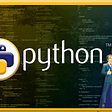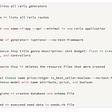# A Simplified Explanation of the Big O Notation

This blog post is a continuation of a series of blog posts about Algorithms, as it has been a hard concept for me to grasp as a programmer. Feel to check out the first blogpost about Algorithms, where I provide an introduction of what Algorithms are and an example of an algorithm and the second blog post about Data Structures, where I explained what are Data Structures and what are some types of Data Structures. Also check out the third blog post about Time Complexity and Space Complexity, which I provide an explanation of Time and Space Complexity.

This blog post I will focus on the Big O Notation.I will explain what is the Big O Notation, how is Big O Notation associated with Algorithms, and provide an some examples.

## What is the Big O Notation?

Big O Notation is the language we use to describe the complexity of an algorithm. In other words, Big O Notation is the language we use for talking about how long an algorithm takes to run. It is how we compare the efficiency of different approaches to a problem. With Big O Notation we express the runtime in terms of — how quickly it grows relative to the input, as the input gets larger.

Let’s break down the last line:

1. how quickly the runtime grows — Being that it is difficult to determine the exact runtime of an algorithm. It depends on the speed of the computer processor. So instead of talking about the runtime directly, we use Big O Notation to talk about how quickly the runtime grows.
2. relative to the input — If we were measuring our runtime directly, we could express our speed in seconds and minutes. Since we are measuring how quickly our runtime grows, we need to express our speed in terms of something else. With Big O Notation, we use the size of the input, which we call “n”. So we can say things like the runtime grows “on the order of the size of the input” (O(n)) or “on the order of the square of the size of the input” (O(n²)).
3. as the input gets larger — Our algorithm may have steps that seem expensive when n is small but are eclipsed eventually by other steps as n gets larger. For Big O Notation analysis, we care more about the stuff that grows fastest as the input grows, because everything else is quickly eclipsed as n gets very large.

## Some Examples of Big O Notation

This function runs in O(1) time (or “constant time”) relative to its input. This means that the input array could be 1 item or 1,000 items, but this function would still just require one “step.”

This function runs in O(n) time (or “linear time”), where n is the number of items in the array. This means that if the array has 10 items, I have to print 10 times. If it has 1,000 items, I have to print 1,000 times.

In this example I am nesting two loops. If the array has n items, the outer loop runs n times and the inner loop runs n times for each iteration of the outer loop, giving us n²​​ total prints. Thus this function runs in O(n²) time (or “quadratic time”). If the array has 10 items, I have to print 100 times. If it has 1,000 items, I have to print 1,000,000 times.

## Example where N could be the actual input, or the size of the input:

Both of these functions have O(n) runtime, even though one takes an integer as its input and the other takes an array:

Sometimes n is an actual number that’s an input to the function, and other times n is the number of items in an input array (or an input map, or an input object, etc.). This means that N could be the actual input, or the size of the input.

## Worst Case Scenario:

When it comes to the Big O Notation, we are usually talking about the worst case scenario. At times the worst case runtime is significantly worse than the best case runtime.

In this example, I might have 100 items in the haystack, but the first item might be the needle, in which case I would return in just 1 iteration of the loop. I can say this is O(n) runtime and the worst case scenario would be implied. But to be more specific I could say this is worst case O(n) and best case O(1) runtime.

## Example of Space Complexity:

There may be times when I want to optimize for using less memory instead of (or in addition to) using less time. Talking about memory cost (or “space complexity”) is very similar to talking about time cost. I simply look at the total size (relative to the size of the input) of any new variables I am allocating.

This function takes O(1) space (I am not allocating any new variables):

This function takes O(n) space (the size of helloArray scales with the size of the input):

Most of the time when we talk about space complexity, we’re talking about additional space, so we don’t include space taken up by the inputs. For example, this function takes constant space even though the input has n items:

At times there can be tradeoff between saving time and saving space, so it is up to you to decide which one you are optimizing for.

Overall Big O Notation is a language we use to describe the complexity of an algorithm. Big O Notation provides approximation of how quickly space or time complexity grows relative to input size. With Big O Notation, we are usually talking about worst case scenario. If you would like to learn more about Big O Notation and build your confidence with Algorithm questions I would recommend checking out Interview Cake. In fact, Interview Cake has a great article about Big O Notation, which was a tremendous resources for me and helped me build my confidence with Big O Notation. And I borrowed their examples as well for this blog post.

--

--

--

## More from Karuna Sehgal

Woman on a mission — to live the best life possible!! A Full Stack Web Developer

## A Review Of PRINTER ISSUES IN WINDOWS 10## The Curious Case of SQL TRUE## CS371p Spring 2021: Erica Martinez## AttributeError: module ‘tensorflow.python.framework.ops’## How 3 Process Patterns will change the way you do Test Automation## 10 Best Project-Based Tutorials To Learn Full-Stack Development## How to Connect to Private AWS Resources with SSH Tunnels and Bastion Hosts## Career Opportunities in Python## Karuna Sehgal

Woman on a mission - to live the best life possible!!

## Pair Programming## Full-Stack Software Engineer## Aiming for object-oriented design elegance